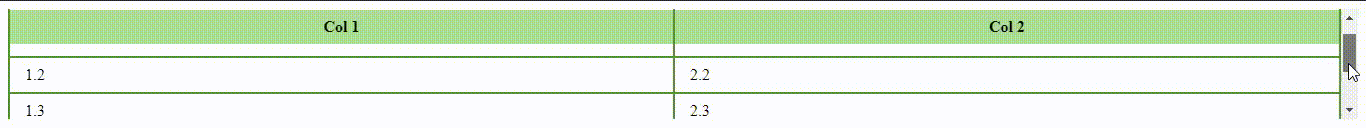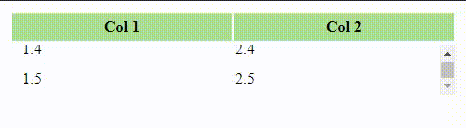# How to create a table with fixed header and scrollable body ?

1. By setting the position property to “sticky” and specifying “0” as a value of the top property for the <th> element.
2. By setting the display to “block” for both <thead> and <tbody> element so that we can apply the height and overflow properties to <tbody>.

Note:

• The position property states the type of positioning for an element.
• The display property states that how an element should be displayed.

Example: Below is the code which illustrates the formation of table with position property.

## HTML

 ` ` `<``html``> ` ` `  `<``head``> ` `  ``<``style``> ` `    ``.fixTableHead { ` `      ``overflow-y: auto; ` `      ``height: 110px; ` `    ``} ` `    ``.fixTableHead thead th { ` `      ``position: sticky; ` `      ``top: 0; ` `    ``} ` `    ``table { ` `      ``border-collapse: collapse;         ` `      ``width: 100%; ` `    ``} ` `    ``th, ` `    ``td { ` `      ``padding: 8px 15px; ` `      ``border: 2px solid #529432; ` `    ``} ` `    ``th { ` `      ``background: #ABDD93; ` `    ``} ` `  `` ` ` ` ` `  `<``body``> ` `  ``<``div` `class``=``"fixTableHead"``> ` `    ``<``table``> ` `      ``<``thead``> ` `        ``<``tr``> ` `          ``<``th``>Col 1 ` `          ``<``th``>Col 2 ` `        `` ` `      `` ` ` `  `      ``<``tbody``> ` `        ``<``tr``> ` `          ``<``td``>1.1 ` `          ``<``td``>2.1 ` `        `` ` `        ``<``tr``> ` `          ``<``td``>1.2 ` `          ``<``td``>2.2 ` `        `` ` `        ``<``tr``> ` `          ``<``td``>1.3 ` `          ``<``td``>2.3 ` `        `` ` `        ``<``tr``> ` `          ``<``td``>1.4 ` `          ``<``td``>2.4 ` `        `` ` `        ``<``tr``> ` `          ``<``td``>1.5 ` `          ``<``td``>2.5 ` `        `` ` `      `` ` `       `  `    `` ` `  `` ` ` ` ` `  ``

Output:Example 2: Below is the code which illustrates the formation of table with display property.

## HTML

 ` ` `<``html``> ` ` `  `<``head``> ` `  ``<``style``> ` `    ``.tableFixHead { ` `      ``width: 500px; ` `      ``table-layout: fixed; ` `      ``border-collapse: collapse; ` `    ``} ` `      ``.tableFixHead tbody { ` `      ``display: block; ` `      ``width: 100%; ` `      ``overflow: auto; ` `      ``height: 50px; ` `    ``} ` `    ``.tableFixHead thead tr { ` `      ``display: block; ` `    ``} ` `    ``.tableFixHead th, ` `    ``.tableFixHead  td { ` `      ``padding: 5px 10px; ` `      ``width: 200px; ` `    ``} ` `    ``th { ` `      ``background: #ABDD93; ` `    ``} ` `  `` ` ` ` ` `  `<``body``> ` `  ``<``div` `class``=``"tableFixHead"``> ` `    ``<``table``> ` `      ``<``thead``> ` `        ``<``tr``> ` `          ``<``th``>Col 1 ` `          ``<``th``>Col 2 ` `        `` ` `      `` ` `      ``<``tbody``> ` `        ``<``tr``> ` `          ``<``td``>1.1 ` `          ``<``td``>2.1 ` `        `` ` `        ``<``tr``> ` `          ``<``td``>1.2 ` `          ``<``td``>2.2 ` `        `` ` `        ``<``tr``> ` `          ``<``td``>1.3 ` `          ``<``td``>2.3 ` `        `` ` `        ``<``tr``> ` `          ``<``td``>1.4 ` `          ``<``td``>2.4 ` `        `` ` `        ``<``tr``> ` `          ``<``td``>1.5 ` `          ``<``td``>2.5 ` `        `` ` `      `` ` `    `` ` `  `` ` ` ` ` `  ``

Output:Whether you're preparing for your first job interview or aiming to upskill in this ever-evolving tech landscape, GeeksforGeeks Courses are your key to success. We provide top-quality content at affordable prices, all geared towards accelerating your growth in a time-bound manner. Join the millions we've already empowered, and we're here to do the same for you. Don't miss out - check it out now!

Previous
Next